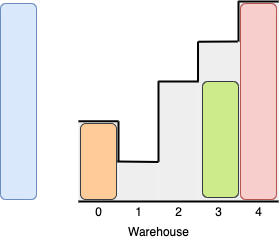Formatted question description: https://leetcode.ca/all/1580.html

# 1580. Put Boxes Into the Warehouse II

Medium

## Description

Given two arrays of positive integers boxes and warehouse representing the heights of some boxes of unit width, and the heights of n rooms in a warehouse, respectively. The warehouse’s rooms are labeled from 0 to n - 1 from left to right where warehouse[i] (0-indexed) is the height of the i-th room.

Boxes are put into the warehouse by the following rules:

• Boxes can’t be piled up.
• You can rearrange the order of the boxes.
• Boxes can only be pushed into the warehouse from either side (left or right).
• If the height of some room in the warehouse is less than the height of a box, then that box and all other boxes behind it will be stopped before that room.

Return the maximum number of boxes you can put into the warehouse.

Example 1:Input: boxes = [1,2,2,3,4], warehouse = [3,4,1,2]

Output: 4

8*Explanation:**We can store the boxes in the following order:

1- Put the yellow box in room 2 from either the left or right side.

2- Put the orange box in room 3 from the right side.

3- Put the green box in room 1 from the left side.

4- Put the red box in room 0 from the left side.

Notice that there are other valid ways to put 4 boxes such as swapping the red and green boxes or the red and orange boxes.

Example 2:Input: boxes = [3,5,5,2], warehouse = [2,1,3,4,5]

Output: 3

Explanation:It’s not possible to put the two boxes of height 5 in the warehouse since there’s only 1 room of height >= 5.

Other valid solutions are to put the green box in room 2 or to put the orange box first in room 2 before putting the green and red boxes.

Example 3:

Input: boxes = [1,2,3], warehouse = [1,2,3,4]

Output: 3

Example 4:

Input: boxes = [4,5,6], warehouse = [3,3,3,3,3]

Output: 0

Constraints:

• n == warehouse.length
• 1 <= boxes.length, warehouse.length <= 10^5
• 1 <= boxes[i], warehouse[i] <= 10^9

## Solution

Since boxes can be pushed into the warehouse from either side, the maximum valid height of each room should be calculated from both sides, and the maximum valid height is the maximum of the two valid heights. Create a new array validWarehouse with length n, where validWarehouse[i] is the maximum valid height of each room (from both sides) for 0 <= i < n.

Sort boxes and validWarehouse. Loop over both arrays in forward direction. For each box, find the room with the minimum height that can contain the box, and put the box into the room. Since all the remaining boxes have equal height or greater height than the current box, only the rooms with equal height or greater height are considered for the remaining boxes. Repeat the process until all the boxes are put into the warehouse or all the valid rooms in the warehouse are used. Finally, return the number of boxes that are put into the warehouse.

class Solution {
public int maxBoxesInWarehouse(int[] boxes, int[] warehouse) {
int roomsCount = warehouse.length;
int[] validWarehouse = new int[roomsCount];
int leftValid = Integer.MAX_VALUE;
for (int i = 0; i < roomsCount; i++) {
leftValid = Math.min(leftValid, warehouse[i]);
validWarehouse[i] = Math.max(validWarehouse[i], leftValid);
}
int rightValid = Integer.MAX_VALUE;
for (int i = roomsCount - 1; i >= 0; i--) {
rightValid = Math.min(rightValid, warehouse[i]);
validWarehouse[i] = Math.max(validWarehouse[i], rightValid);
}
int maxBoxes = 0;
Arrays.sort(boxes);
Arrays.sort(validWarehouse);
int boxesCount = boxes.length;
for (int i = 0, j = 0; i < boxesCount && j < roomsCount; i++) {
while (j < roomsCount && boxes[i] > validWarehouse[j])
j++;
if (j < roomsCount && boxes[i] <= validWarehouse[j]) {
maxBoxes++;
j++;
}
}
return maxBoxes;
}
}